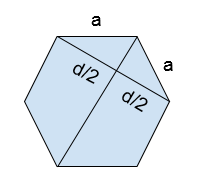# Find length of Diagonal of Hexagon in C++

In this problem, we are given an integer n denoting the length of the side of a regular hexagon. Our task is to Find length of Diagonal of Hexagon.

Problem Description: Here, we have the side of a regular hexagon. And we need to find the length of the diagonal of the hexagon.

Let’s take an example to understand the problem,

Input: a = 7

Output: 12.11

## Solution Approach

To solve the problem and find the length of diagonal which is given by the mathematical formula,

Diagonal = 1.73 * a

Let’s derive the formula,

Here, we have a regular polygon of length a.The angle between the diagonal and side is 600.

The ratio of (d/2)/a is equal to sin 60o

Sin 60o = d/ 2*a

0.866 = d/ 2*a

d = 0.866 * 2 * a

d = 1.73 * a

## Example

Live Demo

#include <iostream>
using namespace std;

int main() {

float a = 12;
float d = 1.73 * a;
cout<<"The length of diagonal is "<<d;
return 0;
}

## Output

The length of diagonal is 20.76